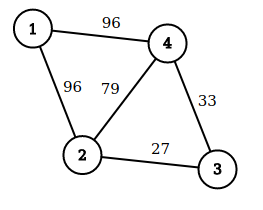# #672. 【UNR #5】航天飞机调度

### 任务

long long solve(int n, int x, int y, int q, std::vector<int> P, int L)

long long distance(int p, int q)

### 样例一

#### input

4 4 1 3 2000000
1 2 96
2 3 27
3 4 33
4 1 96
2 4 79
2
4
4
4

#### output

189

#### explanation• 第一次客流紧张事件发生在 $2$ 号机场。将停留在 $1$ 号机场的飞机调度至 $2$ 号机场，此时调度路线会是 $1 \rightarrow 2$，价值和为 $96$；
• 第二次客流紧张事件发生在 $4$ 号机场。将停留在 $3$ 号机场的飞机调度至 $4$ 号机场，此时调度路线会是 $3 \rightarrow 4$，价值和为 $33$；
• 第三次客流紧张事件依然发生在 $4$ 号机场。将之前停留在 $1$ 号机场、经过第一次客流紧张事件后调度到 $2$ 号机场的飞机调度至 $4$ 号机场，此时调度路线会是 $2 \rightarrow 3 \rightarrow 4$，价值和为 $60$；
• 第四次客流紧张事件仍然发生在 $4$ 号机场。此时两架飞机都停靠在 $4$ 号机场，调度其中任意一架都不会经过额外的航线，价值为 $0$。

• 每一次客流紧张事件结束后，解决该次客流紧张事件的飞机会停靠在客流紧张事件发生的机场，而不是初始机场，如第一次客流紧张事件后原来停留在 $1$ 号机场的飞机停留在了 $2$ 号机场。
• 方案中将某架飞机调度到当前的客流紧张机场时一定会选择经过的航线价值和最小的路径。例如第三次客流紧张事件，将 $2$ 号机场的飞机调度到 $4$ 号机场的调度路线改为 $2 \rightarrow 4$ 是不合法的。
• 方案中不能存在将某一架飞机调度到当前的非客流紧张机场的调度。
• 第三次客流紧张事件发生时，发生事件的 $4$ 号机场已经有一架空闲飞机。此时将另一架飞机调度到 $4$ 号机场是合法的；
• 同时，将一架空闲飞机调度到其所在的机场是合法的，如第四次客流紧张事件的调度。此时经过的航线价值和为 $0$；

### 数据范围

$1$ $20$ $2 \times 10^6$ A $10$
$2$ $400$ $15$
$3$ $1998$
$4$ $3 \times 10^4$ A $10$
$5$ $6 \times 10^4$ B $25$
$6$ $2 \times 10^6$

• 航线集合随机生成，生成方式为：对一个凸多边形，如果点数不超过 3 则退出；否则随机两个不相邻的顶点，在它们之间连边，并对该边分割的两个凸多边形递归处理。
• 每条航线的价值在 $[1,10^9]$ 内独立均匀随机。
• $q$ 次客流紧张事件发生的机场编号在 $[1,n]$ 内独立均匀随机。

• $x=y=1$；
• $q$ 次客流紧张事件发生的机场编号按照时间顺序单调不降，即 solve 函数传入的 P 数组单调不降。